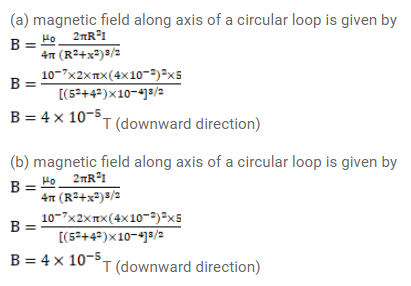# A circular loop of radius 4.0cm is placed in a horizontal

Question:

A circular loop of radius $4.0 \mathrm{~cm}$ is placed in a horizontal plane and carries an electric current of $5.0 \mathrm{~A}$ in the clockwise direction as seen from above. Find the magnetic field

(a) at a point $3.0 \mathrm{~cm}$ above the center of the loop.

(b) At a point $3.0 \mathrm{~cm}$ below the center of the loop.

Solution: